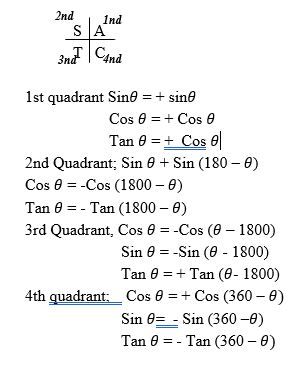Categories

# Trigonometry ratio of Angles – Quadrants

The trigonometry ratio of angles between O0 and 360 are summarized below